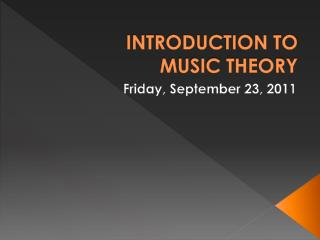DownloadDownload PresentationINTRODUCTION TO MUSIC THEORY

# INTRODUCTION TO MUSIC THEORY

Download Presentation## INTRODUCTION TO MUSIC THEORY

- - - - - - - - - - - - - - - - - - - - - - - - - - - E N D - - - - - - - - - - - - - - - - - - - - - - - - - - -
##### Presentation Transcript

1. INTRODUCTION TO MUSIC THEORY Friday, September 23, 2011

2. TODAY: • Music Sharing – Jonathan • Review: Circle of Fifths • Review: [Generic] Intervals • Introduce: Perfect & Major Intervals • Introduce: Minor Intervals • Introduce: Compound Intervals • RQ8 • For Monday: L35 & L36 • For Monday: Composition on Noteflight!

3. [GENERIC] INTERVALS: • An interval is the distance between two pitches. • The interval is counted from the lower note to the higher note, with the lower note counted as 1 (count every line and space going up the staff). • Intervals are names by the number of the upper note (2nd, 3rd, 4th, 5th, 6th, 7th) with two exceptions: • The interval that is between notes that are identical is called a UNISON (unis.) (or a prime).

4. [GENEREIC] INTERVALS: • An interval of an 8th is called an octave (8va). • Melodic intervals are sounded separately. • Harmonic intervals are sounded together. • Even numbered intervals (2nd, 4th, 6th, 8va) are written from line to space or space to line. • Odd numbered intervals (unis., 3rd, 5th, 7th) are written from line to line or space to space. • Two note combinations are also known as dyads.

5. PERFECT & MAJOR INTERVALS: • Perfect Intervals: • Unison • 4th • 5th • Octave • Major Intervals: • 2nd • 3rd • 6th • 7th

6. DIATONIC INTERVALS:MAJOR SCALE • When the tonic and the upper note of an interval are from the same major scale, it is called a diatonic interval. • All diatonic intervals in the major scale are either perfect (P) or major (M). • This is true for ALL major scales. • P1 = perfect unison • P8 = perfect octave • P1, M2, M3, P4, P5, M6, M7, P8

7. MINOR INTERVALS: • When the interval between the two notes of a major interval (2nd, 3rd, 6th, or 7th) is decreased by a HALF step, it becomes a minor interval. • A small letter “m” is used to signify a minor interval. • Only major intervals can be made into minor intervals; perfect intervals cannot.

8. MORE ABOUT INTERVALS: • Compound intervals are intervals that are larger than an octave are called compound intervals. • A melodic (horizontal) interval is two pitches sounded successively (one after the next). • A harmonic (vertical) interval is two pitches sounded simultaneously.

9. INTERVAL CARNIVAL: • Perfect: Unison (unis.), 4th, 5th, octave (8va) • Major: 2nd, 3rd, 6th, 7th • Minor: 2nd, 3rd, 6th, 7th • Augmented: unis., 2nd, 3rd, 4th, 5th, 6th, 7th, 8va • Diminished: 2nd, 3rd, 4th, 5th, 6th, 7th, 8va